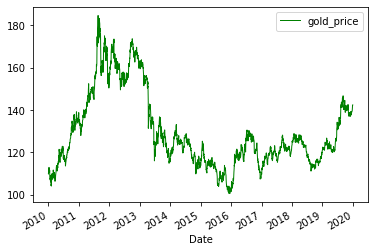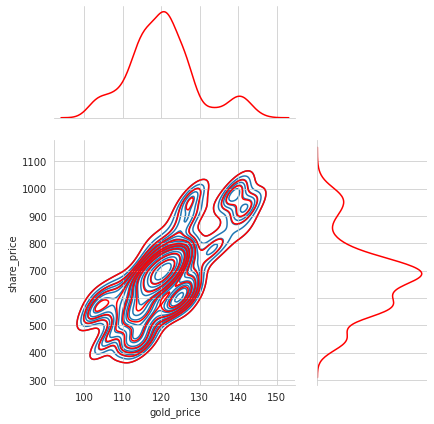As a basis, I took a notebook published on colab for oil. This notebook examines the analysis of gold prices and shares of gold mining companies using machine analysis methods: linear regression, cluster analysis, and random forest. I immediately warn you that this post does not attempt to show the current situation and predict the future direction. Just like the author for oil, this article does not aim to raise or refute the possibilities of machine learning for analyzing stock prices or other tools. I upgraded the code for gold research in order to encourage those who are interested in further reflection and listen to constructive criticism in their address.

```import yfinance as yf
import pandas as pd
import numpy as np
import seaborn as sns
from sklearn import metrics
import matplotlib.pyplot as plt
from sklearn.preprocessing import MinMaxScaler
from sklearn.linear_model import LinearRegression
```

For the price of gold, take the value of the exchange-traded investment Fund SPDR Gold Trust, whose shares are 100% backed by precious metal. The quotes will be compared with the prices of gold mining companies ' shares:

• Newmont Goldcorp (NMM)
• Barrick Gold (GOLD)
• AngloGold Ashanti (AU)
• Kinross Gold (KGC)
• Newcrest Mining (ENC)
• Polyus (PLZL)
• Polymetal (POLY)
• Seligdar (SELG)
```gold = pd.DataFrame(yf.download("GLD", start="2010-01-01", end="2019-12-31")['Adj Close'])
```
```[*********************100%***********************]  1 of 1 completed
```
```gold = gold.reset_index()
gold.columns = ["Date","gold_price"]
gold['Date'] = pd.to_datetime(gold['Date'])
```
Date gold_price
0 2010-01-04 109.800003
1 2010-01-05 109.699997
2 2010-01-06 111.510002
3 2010-01-07 110.820000
4 2010-01-08 111.370003

It is necessary to move the price of gold, as we will be interested in how yesterday's price affected today's stock price.

```gold["gold_price"] = gold["gold_price"].shift(1)
```
```shares=["NMM.SG","GOLD","AU","KGC","NCM.AX","PLZL.ME","POLY.ME","SELG.ME"]
```
```[*********************100%***********************]  8 of 8 completed
```
```data = data.reset_index()
```
Date AU GOLD KGC NCM.AX NMM.SG PLZL.ME POLY.ME SELG.ME
0 2010-01-04 39.698944 34.561649 18.105721 33.237167 26.924570 NaN NaN NaN
1 2010-01-05 40.320408 34.989510 18.594805 33.901924 27.116940 NaN NaN NaN
2 2010-01-06 41.601028 35.733963 19.256504 33.901924 27.289278 NaN NaN NaN
3 2010-01-07 41.130215 35.229092 19.352404 34.298923 NaN NaN NaN NaN
4 2010-01-08 41.601028 35.451572 19.601744 33.421829 27.702093 NaN NaN NaN
```data['Date'] = pd.to_datetime(data['Date'])
```
```all_data=pd.DataFrame()
```
```for index in range(len(shares)):
stock=pd.DataFrame()
# transform the data
stock=data.loc[:, ("Date",shares[index])]
stock["Date"]=stock["Date"].astype('datetime64[ns]')
stock.columns=["Date","share_price"]
test=pd.DataFrame(gold)
output=stock.merge(test,on="Date",how="left") #combining two data sets
stock["gold_price"]=output["gold_price"]
stock['share_price']=pd.to_numeric(stock['share_price'], errors='coerce').dropna(0)
stock['gold_price']=pd.to_numeric(stock['gold_price'], errors='coerce').dropna(0)
stock["year"]=pd.to_datetime(stock["Date"]).dt.year #Create a column with years for subsequent filtering
stock["name"]=shares[index]
stock = stock.dropna() #delete all NAN lines
#creating a column with a scaled share price
scaler=MinMaxScaler()
stock["share_price_scaled"]=scaler.fit_transform(stock["share_price"].to_frame())
#add data to the main dataframe
all_data=all_data.append(stock) #add the data
```
```all_data_15 = all_data[(all_data['year']>2014)&(all_data['year']<2020)]
```
Date share_price gold_price year name share_price_scaled
1301 2015-01-02 14.269927 113.580002 2015 NMM.SG 0.052072
1302 2015-01-05 14.845476 114.080002 2015 NMM.SG 0.071190
1303 2015-01-06 15.601913 115.800003 2015 NMM.SG 0.096317
1304 2015-01-07 15.645762 117.120003 2015 NMM.SG 0.097773
1305 2015-01-08 15.517859 116.430000 2015 NMM.SG 0.093525

## 2. Data analysis

It is best to start analyzing data by presenting it visually, which will help you understand it better.

### 2.1 Chart of gold price changes

```gold[['Date','gold_price']].set_index('Date').plot(color="green", linewidth=1.0)
plt.show()
```### 2.2. Plotting the pairplot chart for the price of Polyus and Barrick Gold shares over the past five years

```palette=sns.cubehelix_palette(18, start=2, rot=0, dark=0, light=.95, reverse=False)
g = sns.pairplot(all_data[(all_data['name']=="POLY.ME")&(all_data['year']>2014)&(all_data['year']<2020)].\
drop(["share_price_scaled"],axis=1), hue="year",height=4)
g.fig.suptitle("Polyuse", y=1.08)

palette=sns.cubehelix_palette(18, start=2, rot=0, dark=0, light=.95, reverse=False)
f = sns.pairplot(all_data[(all_data['name']=="GOLD")&(all_data['year']>2014)&(all_data['year']<2020)].\
drop(["share_price_scaled"],axis=1), hue="year",height=4)
f.fig.suptitle('Barrick Gold', y=1.08)

plt.show()
```A paired graph allows you to see the distribution of data by showing the paired relationships in the data set and the univariate distribution of data for each variable. You can also use the palette to see how this data changed in different years.

The chart is particularly interesting for 2016 and 2019, as it looks like the price of the Pole stock, Barrick Gold and the price of gold are lined up along the same line. We can also conclude from the distribution charts that the price of gold and stocks moved gradually towards higher values.

### 2.3 Violinplot for the gold price

```plt.figure(figsize=(10,10))
sns.set_style("whitegrid")
palette=sns.cubehelix_palette(5, start=2.8, rot=0, dark=0.2, light=0.8, reverse=False)

sns.violinplot(x="year", y="gold_price", data=all_data_15[["gold_price","year"]],
inner="quart", palette=palette, trim=True)
plt.xlabel("Year")
plt.ylabel("Price gold")

plt.show()
```### 2.4 Violinplot for multiple shares

```sns.catplot(x="year", y="share_price_scaled", col='name', col_wrap=3,kind="violin",
split=True, data=all_data_15,inner="quart", palette=palette, trim=True, height=4, aspect=1.2)
sns.despine(left=True)
```A large fluctuation in gold prices was noted according to the charts in 2016 and 2019. As you can see from the graphs in the following figure, some companies such as Newmont Mining, Barrick Gold, AngloGold Ashanti, Newcrest Mining and Polymetal were also affected. It should also be noted that all prices are marked in the range from 0 to 1 and this may lead to inaccuracies in the interpretation.

Next, we will build distribution charts for one Russian company - Polymetal and one foreign company - Barrick Gold

```sns.jointplot("gold_price", "share_price",data=all_data_15[all_data_15['name']=="POLY.ME"],kind="kde",
height=6,ratio=2,color="red").plot_joint(sns.kdeplot, zorder=0, n_levels=20)

sns.jointplot("gold_price", "share_price",data=all_data_15[all_data_15['name']=="GOLD"],kind="kde",
height=6,ratio=2,color="red").plot_joint(sns.kdeplot, zorder=0, n_levels=20)
plt.show()
```
```/usr/local/lib/python3.6/dist-packages/seaborn/_decorators.py:43: FutureWarning: Pass the following variables as keyword args: x, y. From version 0.12, the only valid positional argument will be `data`, and passing other arguments without an explicit keyword will result in an error or misinterpretation.
FutureWarning
/usr/local/lib/python3.6/dist-packages/seaborn/_decorators.py:43: FutureWarning: Pass the following variables as keyword args: x, y. From version 0.12, the only valid positional argument will be `data`, and passing other arguments without an explicit keyword will result in an error or misinterpretation.
FutureWarning
```It is necessary to pay attention to the distribution of the share price for the two companies and it will become clear that the shape of the density graph is the same for them.

### 2.5 Charts of the dependence of the share price of various companies on the price of gold

```sns.lmplot(x="gold_price", y="share_price_scaled", col="name",ci=None, col_wrap=3,
data=all_data_15, order=1,line_kws={'color': 'blue'},scatter_kws={'color': 'grey'}).set(ylim=(0, 1))

plt.show()
```In fact, you won't be able to see much on these charts, although some stocks seem to have a relationship.

The next step is to try to color the charts depending on the years.

```palette=sns.cubehelix_palette(5, start=2, rot=0, dark=0, light=.95, reverse=False)
sns.lmplot(x="gold_price", y="share_price_scaled",hue="year", col="name",ci=None,
col_wrap=3, data=all_data_15, order=1,palette=palette,height=4).set(ylim=(0, 1))

plt.show()
```